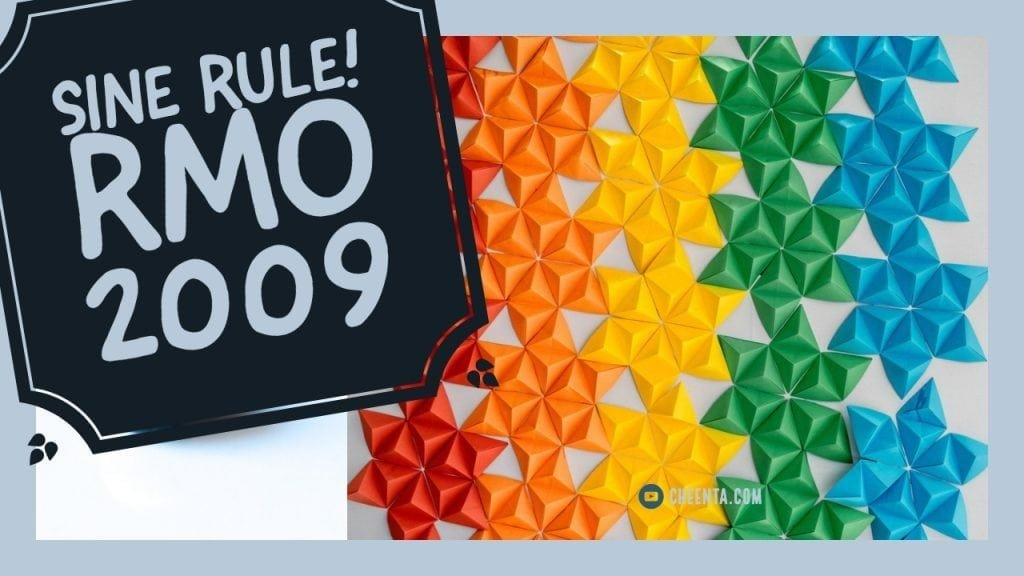How Cheenta works to ensure student success?
Explore the Back-Story

# Sine Rule and Incenter - RMO 2009 Geometry##### The Problem

Let ABC be a triangle in which AB = AC and let I be its in-centre. Suppose BC = AB + AI. Find ∠BAC.

#### Big Ideas

• For any triangle ABC, $\frac{\sin A}{a} = \frac{\sin B } {b} = \frac {\sin C }{c}$.
• Addendo: If $\frac{a}{b} = \frac{c}{d}$ then each of these ratios are equal to $\frac {a+b}{c+d}$
• $\sin (90 + \theta ) = \cos \theta$
• $\cos ^2 2 \theta = 2 \cos ^2 \theta -1 = \cos ^2 \theta - \sin ^2 \theta$

RMO 2009 problems, discussions and other resources. Read more

##### The Problem

Let ABC be a triangle in which AB = AC and let I be its in-centre. Suppose BC = AB + AI. Find ∠BAC.

#### Big Ideas

• For any triangle ABC, $\frac{\sin A}{a} = \frac{\sin B } {b} = \frac {\sin C }{c}$.
• Addendo: If $\frac{a}{b} = \frac{c}{d}$ then each of these ratios are equal to $\frac {a+b}{c+d}$
• $\sin (90 + \theta ) = \cos \theta$
• $\cos ^2 2 \theta = 2 \cos ^2 \theta -1 = \cos ^2 \theta - \sin ^2 \theta$

RMO 2009 problems, discussions and other resources. Read more

This site uses Akismet to reduce spam. Learn how your comment data is processed.

### Knowledge Partner